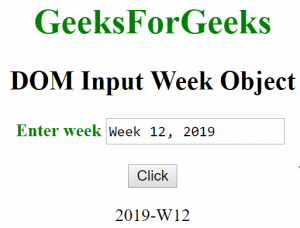Related Articles
HTML | DOM Input Week Object
• Last Updated : 18 Apr, 2019

The Input Week object in HTML DOM represents an <input> element with type = “time” attribute. The element can be accessed by using getElementById() method.

Syntax:

`document.getElementById("id");`

where id is assigned to the <input> tag.

Property Values:

• list: It returns the reference of data list that contains the week field.
• form: It returns the reference of form that contains the week field.
• autocomplete: It is used to set or return the value of the autocomplete attribute of a week field.
• autofocus: It is used to set or return if the week field should automatically get focus when the page loads.
• defaultvalue: It is used set or return the default value of a week field.
• disabled: It is used to set or return whether a week field is disabled or not.
• max: It is used to set or return the value of the max attribute of a week field.
• min: It is used to set or return the value of the min attribute of a week field.
• name: It is used to set or return the value of the name attribute of a week field.
• readOnly: It is used to set or return whether the week field is read-only or not.
• required: It is used to set or return whether the week field must be filled out before submitting a form.
• size: It is used to set or return the value of size attribute of the week field.
• type: It returns the type of form element the week field belongs.
• value: It is used set or return the value of the value attribute of a week field.

Example 1: This example describes the getElementById() method to access the <input> element with type = “week” attribute.

 ` ``<``html``> ``    ``<``head``> ``        ``<``title``>``            ``DOM Input Week Object ``        ````    `` ``     ` `    ``<``body``> ``        ``<``center``> ``            ``<``h1` `style` `= ``"color:green;"``> ``                ``GeeksForGeeks ``            `` ``             ` `            ``<``h2``>DOM Input Week Object ``                 ` `            ``<``label` `for` `= ``"uname"` `style` `= ``"color:green"``>``                ``<``b``>Enter week``            ````             ` `            ``<``input` `type` `= ``"week"` `id` `= ``"gfg"` `                ``placeholder` `= ``"Enter week"``>``             ` `            ``<``br``><``br``>``                 ` `            ``<``button` `type` `= ``"button"` `onclick` `= ``"geeks()"``> ``                ``Click``            `` ``             ` `            ``<``p` `id` `= ``"GFG"``>``             ` `            ``<``script``> ``                ``function geeks() { ``                    ``var link = document.getElementById("gfg").value; ``                    ``document.getElementById("GFG").innerHTML = link;``                ``} ``            `` ``        ````    `` ``                    `

Output:
Before Click on the button:Example 2: This example describes the document.createElement() method to create <input> element with type = “week” attribute.

 ` ``<``html``> ``    ``<``head``> ``        ``<``title``>``            ``DOM Input Week Object ``        ````    `` ``     ` `    ``<``body``> ``        ``<``center``> ``            ``<``h1` `style` `= ``"color:green;"``> ``                ``GeeksForGeeks ``            `` ``             ` `            ``<``h2``>DOM Input Week Object ``                 ` `            ``<``label` `for` `= ``"uname"` `style` `= ``"color:green"``>``                ``<``b``>Enter week``            ````             ` `        ``<``p` `id` `= ``"GFG"``>``             ` `         ` `            ``<``button` `type` `= ``"button"` `onclick` `= ``"geeks()"``> ``                ``Click``            `` ``             ` `            ````            ``<``script``> ``                ``function geeks() {``                     ` `                    ``/* Create input element  */``                    ``var link = document.createElement("INPUT");``                     ` `                    ``/* Set the type attribute */``                    ``link.setAttribute("type", "week");``                     ` `                    ``/* Append node value */``                    ``document.getElementById("GFG").appendChild(link);``                ``} ``            `` ``        ````    `` ``                                          `

Output:
Before Click on the button:Before Click on the button:My Personal Notes arrow_drop_up